Microkit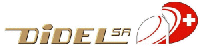Motor specifications

DIDEL proposes several difficult to get motors for the development of microrobots, actuators and Ultra-Light Slow flyers (ULS Ultra-Léger de Salon).  These motors have been carefully measured and documented in order to design an efficient motor/gearbox/propeller system.  Our 4 to 10mm pager motors are well adapted for 2-5 cm microrobots, small actuators and 10-30 grams slow flyers. The MS08 is a powerfull, expensive brand new motor from API-Portescap, for 40-60 grams indoor planes (voltages up to 5V). Didel sells also the switec stepping motor, perfect for light 5-8 cm small robots.  See our price-list, if you are not coming from there.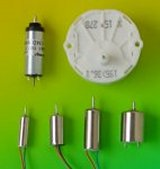This document analyses electric motors below 12mm in diameter and compares their specifications. It provides precise data to those willing to optimize a propeller for a given motor-gearbox, or select a gearbox according to the selected motor and propeller.

Coreless motor theory

Coreless motors have a quite simple model (figure 1). The torque is proportional to the current; the torque constant k, expressed in [mNm/A] depends on the size and design of the motor. At zero speed (stall), the torque Ms [mNm] and the current Is [mA] are at maximum. With the speed, a back-EMF develops until the torque reaches zero, at no-load maximum speed No [RPM]. A current Io [mA] remains to compensate for the friction. The effective power reaches a maximum at half the maximum speed, that is also half the maximum torque. The electrical power is higher due mostly to ohmic dissipation. The efficiency reaches a maximum at a theoretical value of 17% of the No maximum speed, far from the maximum power value we are interested in.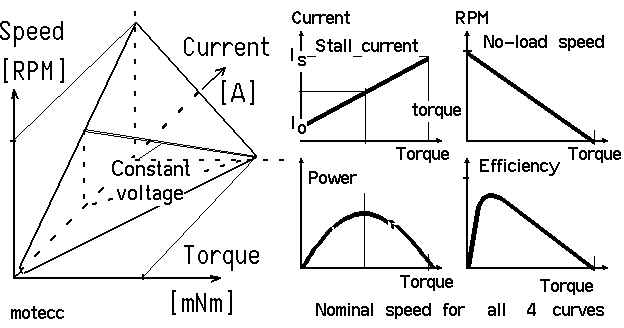Figure 1

The coil resistance R is the other important parameter along with the torque constant. It gives a rough idea of the motor power, for a given voltage. The coil current generates the torque, that is, mechanical power. The coil resistance generates heat that degrades the motor characteristics and may destroy it. The dissipated power is proportional to the square of the current. Overcurrent, due to a blocked motor or overvoltage, results in a quick overheat, and possible destruction of the motor. Over speed is also destructive for the brushes.

The no-load current is related to friction from the sleeves and brushes, and is worse the greater the miniaturization. For instance, Io/Is is 1% for a 26mm Maxon, Minimotor or Portescap motor, 2% for the MS08, 15% for the Mk10, 17% for the MK06 and 30% for the MK04.

Talking about the power of a motor is complicated. Power depends on the voltage, and one can increase the voltage until the motor explodes. Serious manufacturers document a maximum torque, speed and power, taking care of life-time and parameter stability.  The life-time of a motor is specified at the nominal voltage and power (1000 hours, 100 hours for pager motors). The life-time is mostly conditioned by brush wear, which is roughly  inversely proportional to the dissipated power.  Indoor hobbyists are used to overpowering their motors and change the motor or the brushes frequently. We will try to recommend a voltage that allows for a more comfortable lifetime of about 10 hours.

Formulas and test bench

 Couple M [mNm] Electrical power Pel [W] Thermal dissipation Pth [W] Angular speed w [rad/s] Mechanical power Pmec [mW] Max estimated power Pmax [mW] M = k * I   --  k Torque constant [mNm/A]   I Current [A] Pel = U * I   -- U voltage [V] Pth = R * I^2   -- R coil resistance (plus losses due to friction) w = (2*PI/60)*RPM = 0.104 * RPM      -- RPM Revolutions per minute Pmec = w * M = 0.104 * RPM * M Pmax = 1/4 * Ms * No The torque constant is measured from the stall torque. The no-load rotation speed is measured with a small magnet attached to the axis, and a Hall sensor connected to a frequency meter.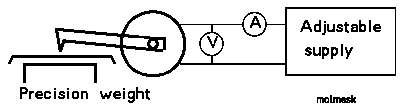Figure 2

Maximum power

The well known linear plot of Figure 3 shows the current and torque as a function of the rotation speed,which is an easy parameter to measure. When the voltage increases, the two curves moves up, and the no-load rotation speed increases. Manufacturers document their motors for a nominal voltage only. Slow flyer motors are over volted in order to get more power for the same weight; a factor of two to three can be applied but the specs degrade when the motor is hot.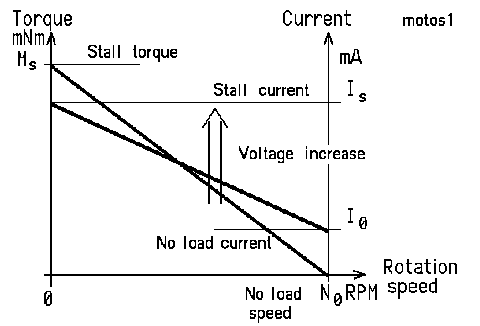Figure 3

Logarithmic plots

Logarithmic scales are efficient to plot small and large values with the same relative precision. Straight lines, except the ones passing by the origin, are transformed into curves, and all curves of the type y = x^n (e.g. a parabola or an hyperbola) appear as straight lines. If we plot the torque as a function of the rotation speed, the constant-power hyperboles are 45 degrees straight lines on a logarithmic plot (same x-y scale).

The transmitted power is maximum at half the stall torque Ms, that is also half the no-load speed No.  We are only interested in the part of the curve around the maximum transmitted power. On the logarithmic plot, the straight line torque vs. RPM becomes a curve that is always the same shape, and the maximum power point moves at 45 degrees when the voltage changes.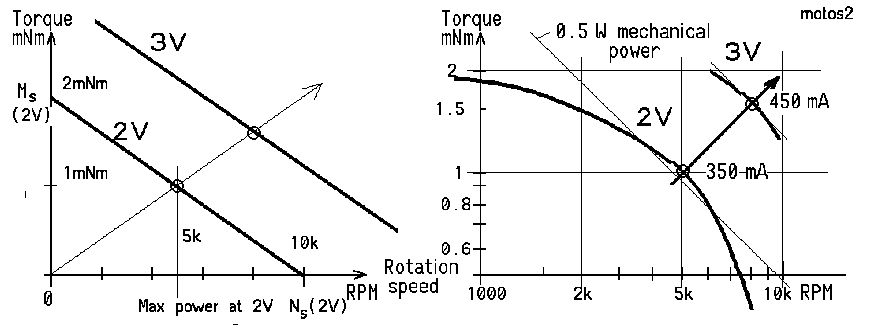Figure 4

 If we add a gearbox to the motor, the curves are just shifted diagonally according to the reduction ratio: a ratio of two, as shown in the figure 5, will decrease the optimal speed by two, and double the torque. The 90% or so efficiency of the gearbox reduces the torque and shifts the curve by a constant distance (not shown on figure).  The current value is given only for the max power. Since around the max power, the current is inversely proportional to the rotations speed, it is easy to estimate it. For example, a 10% increase in RPM will result in a 10% decrease of the current, if the voltage remains the same.  Matching a propeller to a motor with the appropriate gearbox results from these curves. See our propeller report. Figure 5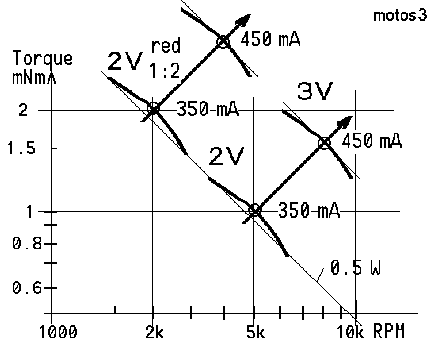DIDEL's motor specifications

For slow flying applications, a motor is mainly characterized by its max power within a voltage range. That power is rougly proportional to the square of the voltage. The power coefficient allows computing the power when the voltage is measured.

The following table surveys the motors measured by DIDEL. Click on the motor number to get the Excel data and graphs if you want to get more details on a given motor. You can also see the Excel file that built figures 6 and 7. Explanations on the column content follows the tables.

The important columns of this table are the max power parameters — which depend on the voltage, as long temperature does not degrade the characteristics at high voltage. At low voltage, friction is also important, but we do not try to work in this range.

 At max power Quality factor Motors Weight Resistance Vnom/Vmax Torque const Power constant Speed const Current const TR Cst M/P*V TRM TR/Weight Figure of merit unit g Ohm V mNm/A mW/V2 RPM/V mA/V MS08-12 4.5 12.6 2 / 5 2.8 18 1690 39 57 12.5 MK10-10 4.8 9.4 1.2 / 4 1.4 22 3400 49 27 5.6 5.6 MK07-08 3.1 7.5 1.2 / 4 1.0 15 3200 50 31 10 10 MK06L-09 2.1 9 1.2 / 4 0.8 24 4500 65 21 10.2 10.2 MK06-10 1.4 10 1.2 / 4 1.1 11 4000 36 16 11.7 11.7 MK06-30 1.4 32 2.5 / 4+ 1.2 5.5 3600 14.5 27 20 20 MK04.24 0.7 24 2.4 /  5 0.75 4.5 6800 15 14 20 20
Figure 6

Not sold by Didel

These motors have been selected to compare with other 6mm page motors, and have a reference point with 12mm motors used on slow flyers.
 At max power Quality factor Motors Dia x L Weight Resistance Vmax Torque const Power constant Speed const Current const TR Cst M/P*V TRM TR/Weight unit mm g Ohm V mNm/A mW/V2 RPM/V mA/V NA06-12 6x12 1.04 12 2.5 0.7 12 5400 38 18 17.3 BC06-03 6x12 1.4 3.1 2 0.5 50 8300 150 11 8.2 A12-04 10x15 3.3 2.3 4 0.65 40 4000 160 42 4.2 Mab12 12x15 5.6 6,6 5 2.4 15 1350 156 71 12.5 DC5-24 12x18 10 4 4-5 2.2 56 1670 150 56 12.5
Figure 7
NA06-12 is a pager motor from Namiki. BC06-4 is the 6mm pager motor from BitCharger. A12 is the A-Max 12mm 2.4V from Maxon. Mab12 is a Mabuchi-like core motor. DC5-2.4 is a coreless from Wes-Technik.

Tables legend

Max power is the best operating point +/- 20% is still acceptable
Power constant. To estimate the power at a given voltage, one multiplies the constant by the square of the voltage.
Speed constant. To estimate the rotation speed at a given voltage, one multiplies the constant by the voltage.
Current constant. To estimate the current at a given voltage, one multiplies the constant by the voltage.
The other columns are more or less evident:
Dia x L Motor diameter and length, not including the shaft and wires (see drawings)
Weight in grams
Coil resistance in Ohms
Nominal voltage given by the manufacturer and estimated overvoltage for a 10h lifetime (no guaranties, systematic tests would imply the destruction of 100 motors)
Torque constant in mNm/A.

Quality factors
How to compare all these motors? We need the maximum torque for the minimum of power. This ratio depends on the voltage, so we divide by V to get the TR (torque ratio) constant. For slow flyers, we give also the TRM constant by dividing by the motor mass. These constants increase with the size of the motor. They change slightly for a given diameter, and depend on the coil resistance, and on the magnet and coil quality.

 The figure of merit of the motors is given in the last column. It is the ratio of the Joule losses, due to the coil resistance, by the power given by the motor. The formula is Merit = k/coil resistance^0.5 (k is the torque constant). This ratio is only 0.14 for 4mm pagers, 0.84 for good coreless motors (MS08 and DC5-24). Larger motors go up to 2 and more, but it's not our game. There may be significant errors on some motors, since frequently only a single motor has been measured. Sorry for that. We will do the correction as soon the errors are discovered.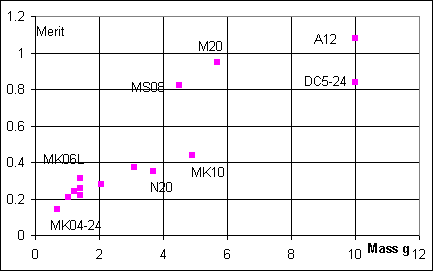Comparison plot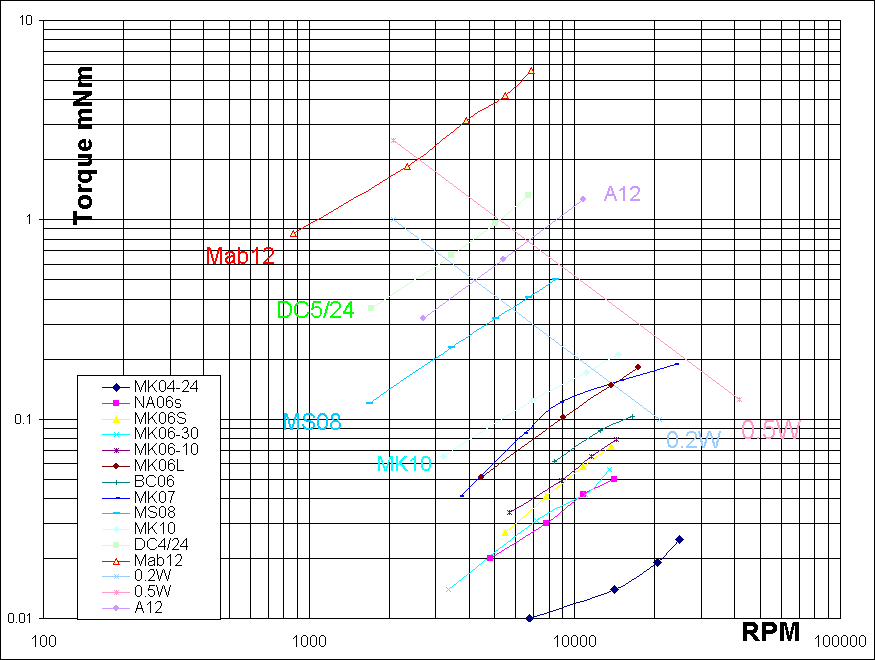Figure 6

If you are concerned about battery weight, the current you need to achieve a given power, that is a given propeller thrust, is your concern. The battery's voltage drop depends on its capacity per element. You cannot drive a DC5-2.4 with a 70 mA set of cells. The Current constant allows estimating the current for a given voltage.

Gearboxes and propellers

See our gearbox section to see what DIDEL proposes as propeller gear trains. If you measure your propeller, you can find the best match.

Note on pager motors

DIDEL gets its pager motors from Jinlong Machinery, Hong-Kong (http://www.kotl.com/). They are very primitively documented. We selected several 4, 6, 7, and 10mm motors, with high resistance coils whenever possible, in order to reduce the consumed current for small robots, or allow a higher battery voltage for slow flyers. These motors are very well built, but one has to understand their construction to avoid any damage to the brushes when assembling. It is not possible to put any axial force on the axes. This is a major problem when inserting gears (see gear insertion recommendations).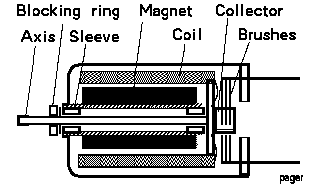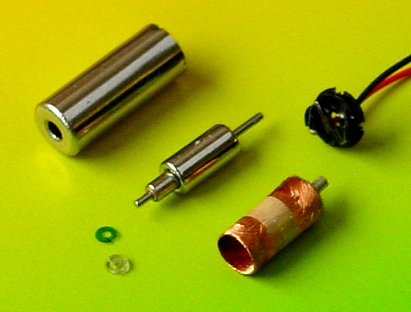CH-1092 Belmont/Lausanne Switzerland Tel +41 21 728-6156 Fax -6157 info@didel.com www.didel.com